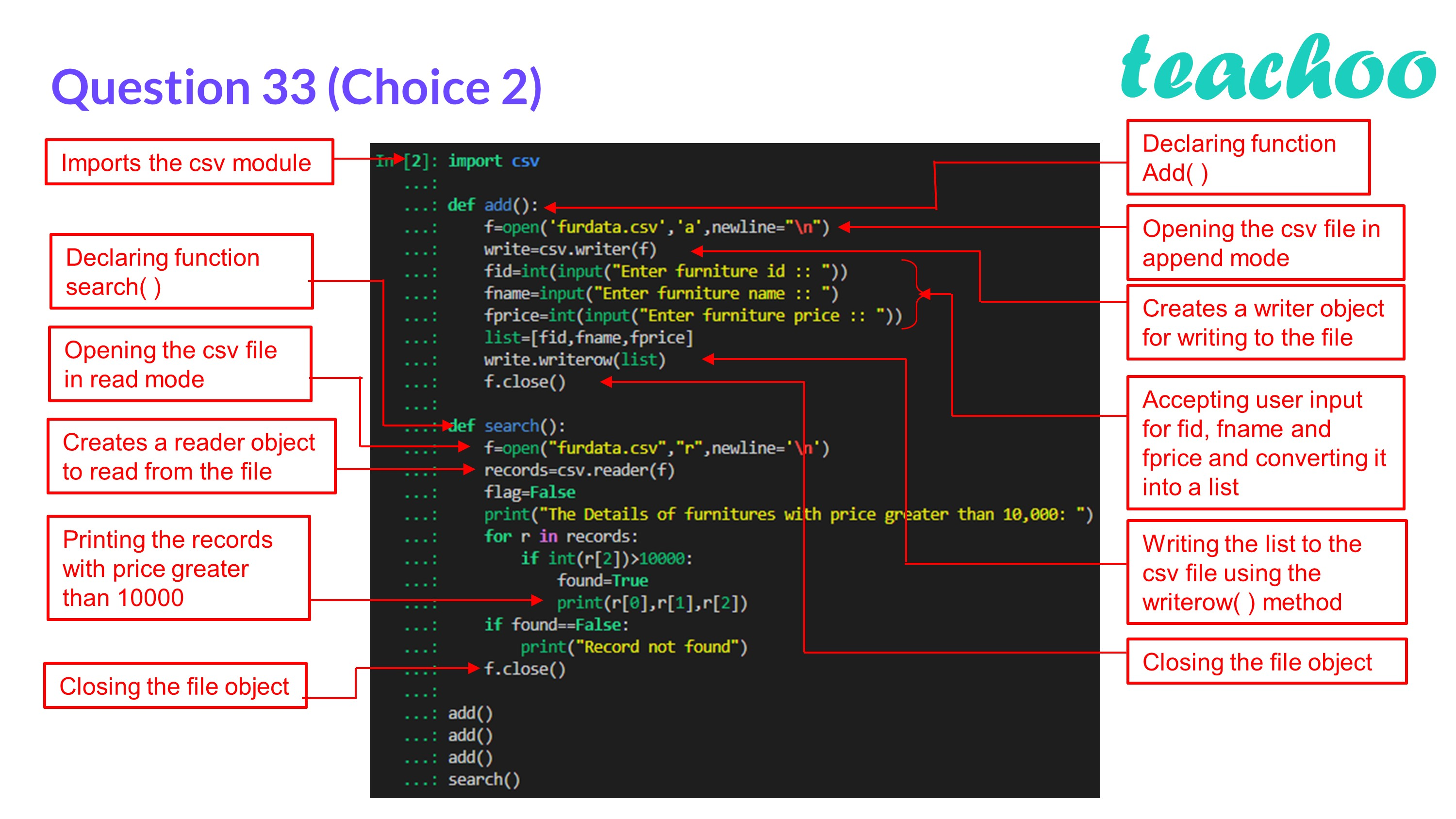CBSE Class 12 Sample Paper for 2023 Boards

Computer Science - Class 12
Solutions to CBSE Sample Paper - Computer Science Class 12

## (ii) COUNTR() – To count the number of records present in the CSV file named ‘record.csv’.

 csv File Binary File Stores information in the form of a text file. Stores information in the form of 0s and 1s. Human readable Not human readable Extension is .csv Extension is .dat

Code:

import csv

def ``` add ``` ():

f=open( ``` 'furdata.csv','a', ``` newline= ``` "\n" ``` )

write=csv.writer(f)

fid=int(input( ``` "Enter furniture id :: " ``` ))

fname=input( ``` "Enter furniture name :: " ``` )

fprice=int(input( ``` "Enter furniture price :: " ``` ))

list=[fid,fname,fprice]

write.writerow(list)

f.close()

def ``` search ``` ():

f=open( ``` "furdata.csv","r" ``` ,newline= ``` '\n' ``` )

flag=False

print( ``` "The Details of furnitures with price greater than 10,000: " ``` )

for r in records:

if int(r[ ``` 2 ``` ])> ``` 10000 ``` :

found=True

print(r[ ``` 0 ``` ],r,r)

if found==False:

print( ``` "Record not found" ``` )

f.close()

search()Learn in your speed, with individual attention - Teachoo Maths 1-on-1 Class